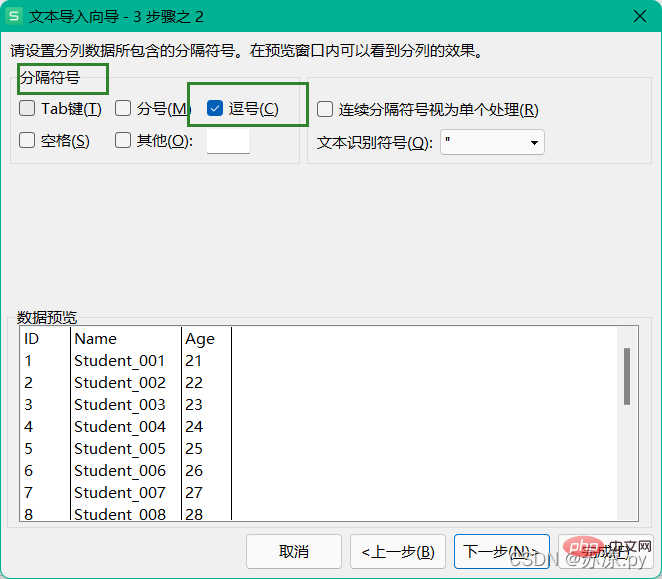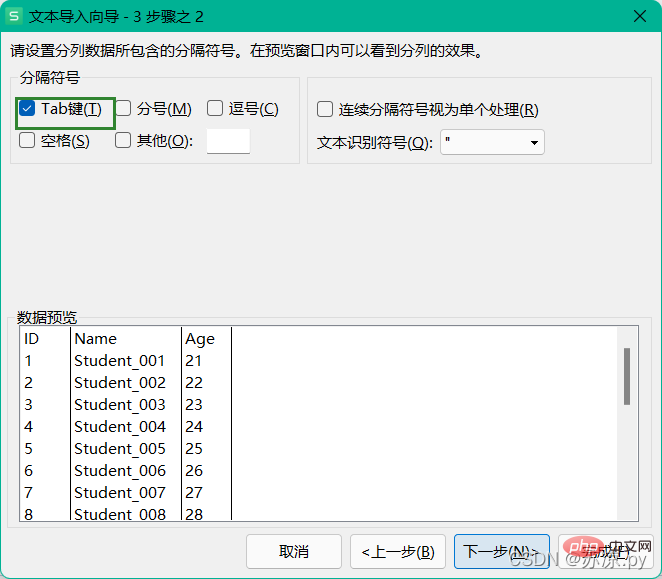# python数据分析之pandas搞定Excel表格

## 1.excel读取其他文件### 1.1 导入csv文件### 1.2 导入tsv文件### 1.3 导入txt文本文件## 2.pandas读取其他文件

### 2.1 读取csv文件

```import pandas as pd

# 导入csv文件
df1 = pd.DataFrame(test1)

print(df1)```

### 2.2 读取tsv文件

tab键用\t来表示

```import pandas as pd

# 导入tsv文件
df3 = pd.DataFrame(test3)

print(df3)```

### 2.3 读取txt文件

```import pandas as pd

# 导入txt文件
df2 = pd.DataFrame(test2)

print(df2)```## 1.在excel中制作透视表## 2.在pandas中绘制透视表

```import pandas as pd
import numpy as np

pd.options.display.max_columns =999
df = pd.DataFrame(test)
# 将年份取出并新建一个列名为年份的列
df['year'] = pd.DatetimeIndex(df['Date']).year
# 绘制透视表
table = df.pivot_table(index='总类',columns='year',values='销售额',aggfunc=np.sum)
df1 = pd.DataFrame(table)
df1['总计'] = df1[[2011,2012,2013,2014]].sum(axis=1)

print(df1)``````import pandas as pd
import numpy as np

pd.options.display.max_columns =999
df = pd.DataFrame(test)
# 将年份取出并新建一个列名为年份的列
df['year'] = pd.DatetimeIndex(df['Date']).year

# groupby方法
group = df.groupby(['总类','year'])

s= group['销售额'].sum()
c = group['ID'].count()

table = pd.DataFrame({'sum':s,'total':c})

print(table)```1/1